Uses a non-parametric approach to reconstruct cases by date of infection from reported cases. It uses either a generative Rt model or non-parametric back calculation to estimate underlying latent infections and then maps these infections to observed cases via uncertain reporting delays and a flexible observation model. See the examples and function arguments for the details of all options. The default settings may not be sufficient for your use case so the number of warmup samples (stan_args = list(warmup)) may need to be increased as may the overall number of samples. Follow the links provided by any warnings messages to diagnose issues with the MCMC fit. It is recommended to explore several of the Rt estimation approaches supported as not all of them may be suited to users own use cases. See here for an example of using estimate_infections within the epinow wrapper to estimate Rt for Covid-19 in a country from the ECDC data source.

## Usage

estimate_infections(
reported_cases,
generation_time = generation_time_opts(),
delays = delay_opts(),
truncation = trunc_opts(),
rt = rt_opts(),
backcalc = backcalc_opts(),
gp = gp_opts(),
obs = obs_opts(),
stan = stan_opts(),
horizon = 7,
CrIs = c(0.2, 0.5, 0.9),
zero_threshold = Inf,
weigh_delay_priors = TRUE,
id = "estimate_infections",
verbose = interactive()
)

## Arguments

reported_cases

A data frame of confirmed cases (confirm) by date (date). confirm must be integer and date must be in date format.

generation_time

A call to generation_time_opts() defining the generation time distribution used. For backwards compatibility a list of summary parameters can also be passed.

delays

A call to delay_opts() defining delay distributions and options. See the documentation of delay_opts() and the examples below for details.

truncation

A call to trunc_opts() defining the truncation of observed data. Defaults to trunc_opts(). See estimate_truncation() for an approach to estimating truncation from data.

rt

A list of options as generated by rt_opts() defining Rt estimation. Defaults to rt_opts(). Set to NULL to switch to using back calculation rather than generating infections using Rt.

backcalc

A list of options as generated by backcalc_opts() to define the back calculation. Defaults to backcalc_opts().

gp

A list of options as generated by gp_opts() to define the Gaussian process. Defaults to gp_opts().Set to NULL to disable the Gaussian process.

obs

A list of options as generated by obs_opts() defining the observation model. Defaults to obs_opts().

stan

A list of stan options as generated by stan_opts(). Defaults to stan_opts(). Can be used to override data, init, and verbose settings if desired.

horizon

Numeric, defaults to 7. Number of days into the future to forecast.

CrIs

Numeric vector of credible intervals to calculate.

Logical, defaults to TRUE. Should zeros at the start of the time series be filtered out.

zero_thresholdNumeric defaults to Inf. Indicates if detected zero cases are meaningful by using a threshold number of cases based on the 7 day average. If the average is above this threshold then the zero is replaced with the backwards looking rolling average. If set to infinity then no changes are made.

weigh_delay_priors

Logical. If TRUE (default), all delay distribution priors will be weighted by the number of observation data points, in doing so approximately placing an independent prior at each time step and usually preventing the posteriors from shifting. If FALSE, no weight will be applied, i.e. delay distributions will be treated as a single parameters.

id

A character string used to assign logging information on error. Used by regional_epinow to assign errors to regions. Alter the default to run with error catching.

verbose

Logical, defaults to TRUE when used interactively and otherwise FALSE. Should verbose debug progress messages be printed. Corresponds to the "DEBUG" level from futile.logger. See setup_logging for more detailed logging options.

## Value

A list of output including: posterior samples, summarised posterior samples, data used to fit the model, and the fit object itself.

epinow regional_epinow forecast_infections simulate_infections

Sam Abbott

## Examples

# \donttest{
# set number of cores to use
old_opts <- options()
options(mc.cores = ifelse(interactive(), 4, 1))

# get example case counts
reported_cases <- example_confirmed[1:60]

# set up example generation time
generation_time <- get_generation_time(
disease = "SARS-CoV-2", source = "ganyani", fixed = TRUE
)
# set delays between infection and case report
incubation_period <- get_incubation_period(
disease = "SARS-CoV-2", source = "lauer", fixed = TRUE
)
# delays between infection and case report, with uncertainty
incubation_period_uncertain <- get_incubation_period(
disease = "SARS-CoV-2", source = "lauer"
)
reporting_delay <- dist_spec(
mean = convert_to_logmean(2, 1), mean_sd = 0,
sd = convert_to_logsd(2, 1), sd_sd = 0, max = 10
)

# default settings but assuming that delays are fixed rather than uncertain
def <- estimate_infections(reported_cases,
generation_time = generation_time_opts(generation_time),
delays = delay_opts(incubation_period + reporting_delay),
rt = rt_opts(prior = list(mean = 2, sd = 0.1)),
stan = stan_opts(control = list(adapt_delta = 0.95))
)
#> Warning: There were 16 divergent transitions after warmup. See
#> https://mc-stan.org/misc/warnings.html#divergent-transitions-after-warmup
#> to find out why this is a problem and how to eliminate them.
#> Warning: Examine the pairs() plot to diagnose sampling problems
# real time estimates
summary(def)
#>                                  measure                estimate
#> 1: New confirmed cases by infection date     2288 (1090 -- 4452)
#> 2:        Expected change in daily cases       Likely decreasing
#> 3:            Effective reproduction no.      0.88 (0.61 -- 1.2)
#> 4:                        Rate of growth -0.026 (-0.099 -- 0.04)
#> 5:          Doubling/halving time (days)          -26 (18 -- -7)
# summary plot
plot(def)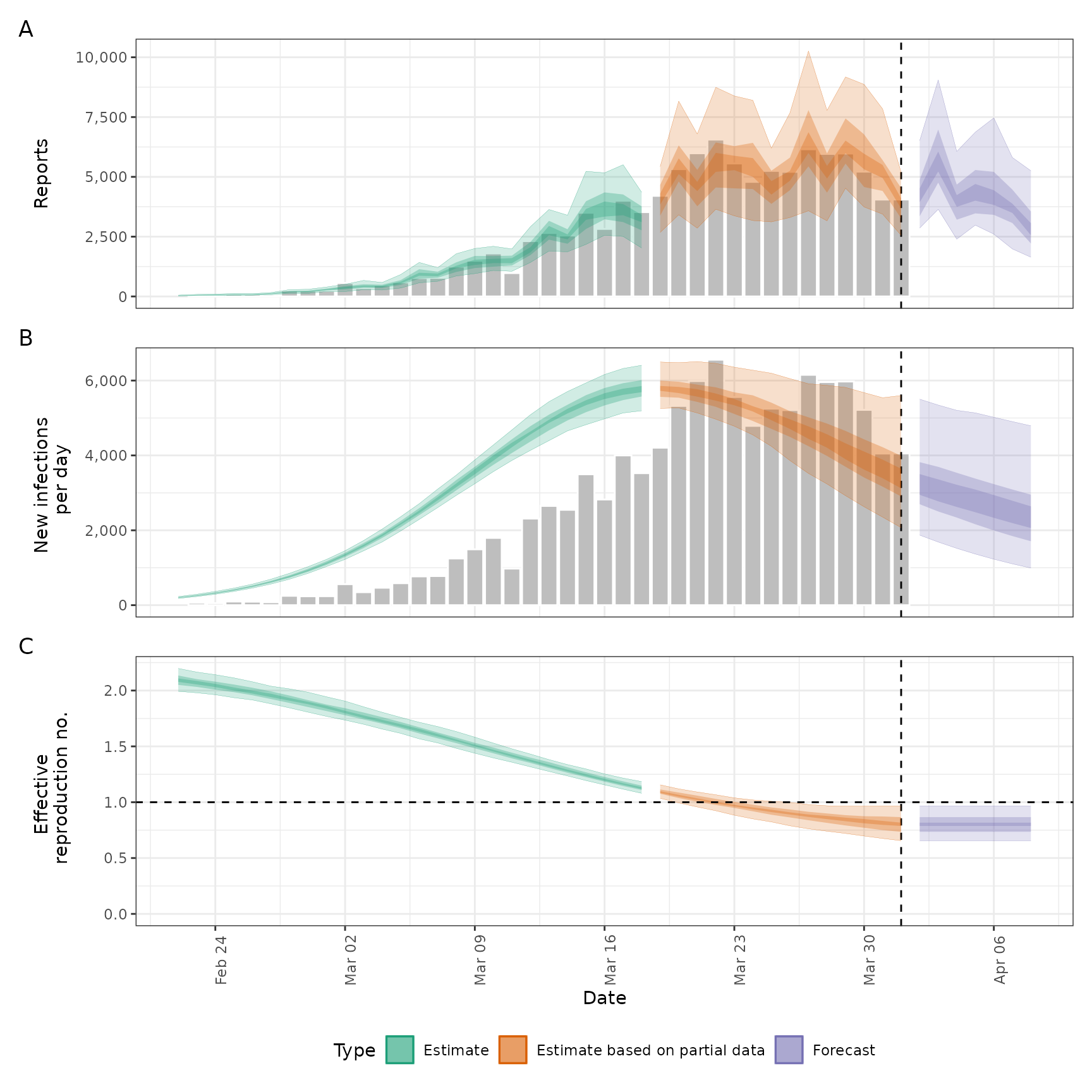# decreasing the accuracy of the approximate Gaussian to speed up
#computation.
# These settings are an area of active research. See ?gp_opts for details.
agp <- estimate_infections(reported_cases,
generation_time = generation_time_opts(generation_time),
delays = delay_opts(incubation_period + reporting_delay),
rt = rt_opts(prior = list(mean = 2, sd = 0.1)),
gp = gp_opts(ls_min = 10, basis_prop = 0.1),
stan = stan_opts(control = list(adapt_delta = 0.95))
)
#> Warning: There were 7 divergent transitions after warmup. See
#> https://mc-stan.org/misc/warnings.html#divergent-transitions-after-warmup
#> to find out why this is a problem and how to eliminate them.
#> Warning: Examine the pairs() plot to diagnose sampling problems
summary(agp)
#>                                  measure                 estimate
#> 1: New confirmed cases by infection date      2317 (1222 -- 4466)
#> 2:        Expected change in daily cases        Likely decreasing
#> 3:            Effective reproduction no.       0.89 (0.65 -- 1.2)
#> 4:                        Rate of growth -0.025 (-0.086 -- 0.039)
#> 5:          Doubling/halving time (days)         -28 (18 -- -8.1)
plot(agp)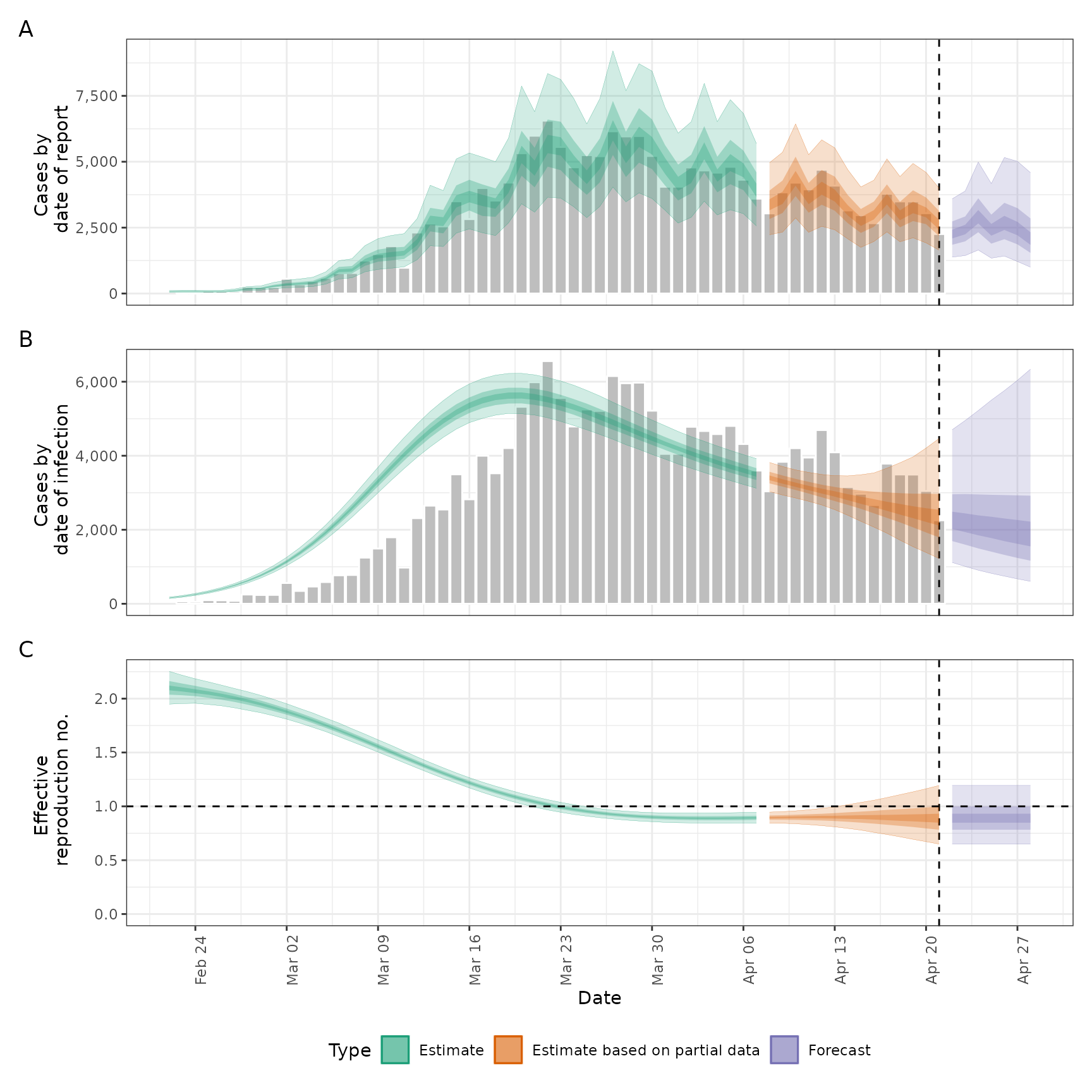# Adjusting for future susceptible depletion
dep <- estimate_infections(reported_cases,
generation_time = generation_time_opts(generation_time),
delays = delay_opts(incubation_period + reporting_delay),
rt = rt_opts(
prior = list(mean = 2, sd = 0.1),
pop = 1000000, future = "latest"
),
gp = gp_opts(ls_min = 10, basis_prop = 0.1), horizon = 21,
stan = stan_opts(control = list(adapt_delta = 0.95))
)
#> Warning: There were 6 divergent transitions after warmup. See
#> https://mc-stan.org/misc/warnings.html#divergent-transitions-after-warmup
#> to find out why this is a problem and how to eliminate them.
#> Warning: Examine the pairs() plot to diagnose sampling problems
plot(dep)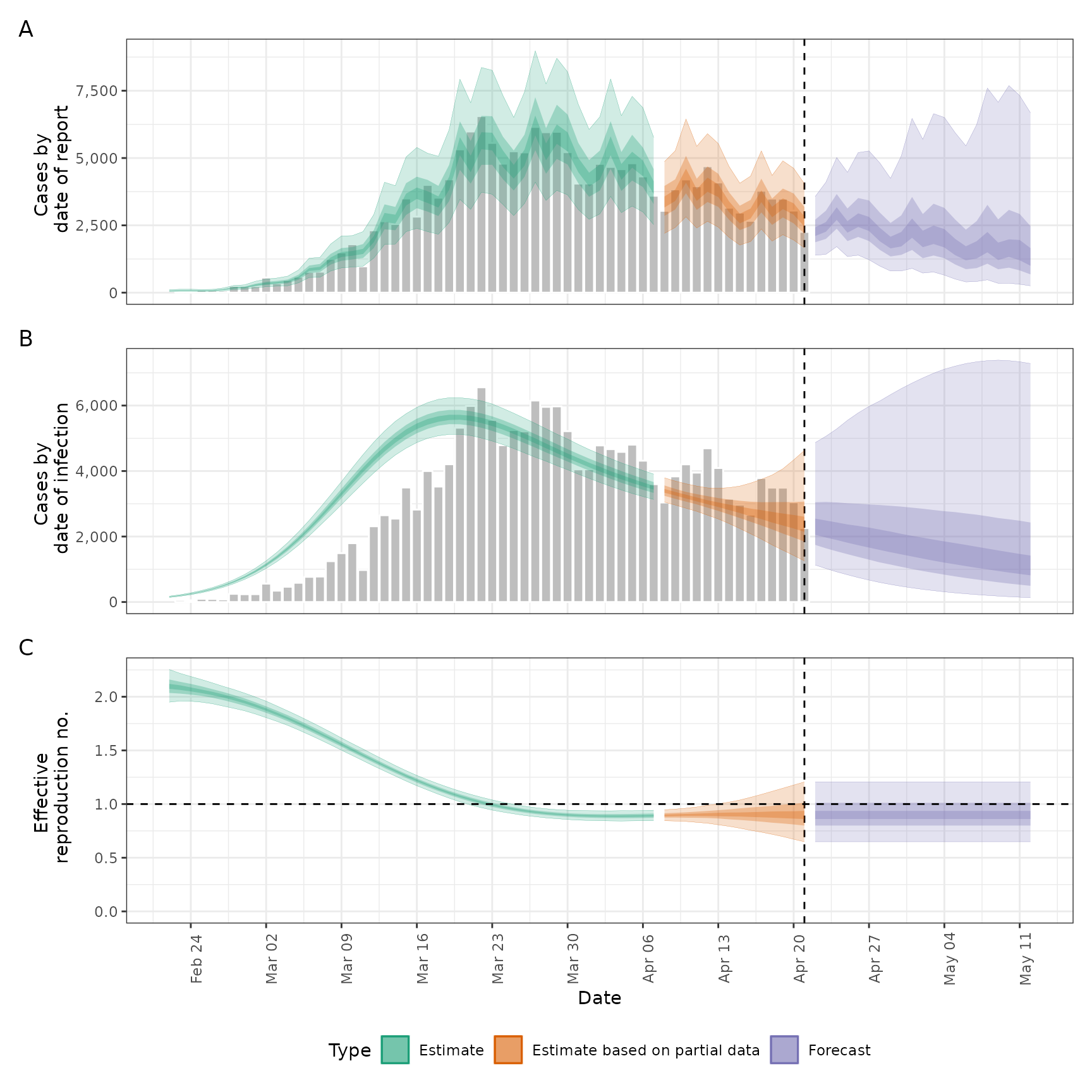# Adjusting for truncation of the most recent data
# See estimate_truncation for an approach to estimating this from data
trunc_dist <- dist_spec(
mean = convert_to_logmean(0.5, 0.5), mean_sd = 0.1,
sd = convert_to_logsd(0.5, 0.5), sd_sd = 0.1,
max = 3
)
trunc <- estimate_infections(reported_cases,
generation_time = generation_time_opts(generation_time),
delays = delay_opts(incubation_period + reporting_delay),
truncation = trunc_opts(trunc_dist),
rt = rt_opts(prior = list(mean = 2, sd = 0.1)),
gp = gp_opts(ls_min = 10, basis_prop = 0.1),
stan = stan_opts(control = list(adapt_delta = 0.95))
)
#> Warning: There were 12 divergent transitions after warmup. See
#> https://mc-stan.org/misc/warnings.html#divergent-transitions-after-warmup
#> to find out why this is a problem and how to eliminate them.
#> Warning: Examine the pairs() plot to diagnose sampling problems
plot(trunc)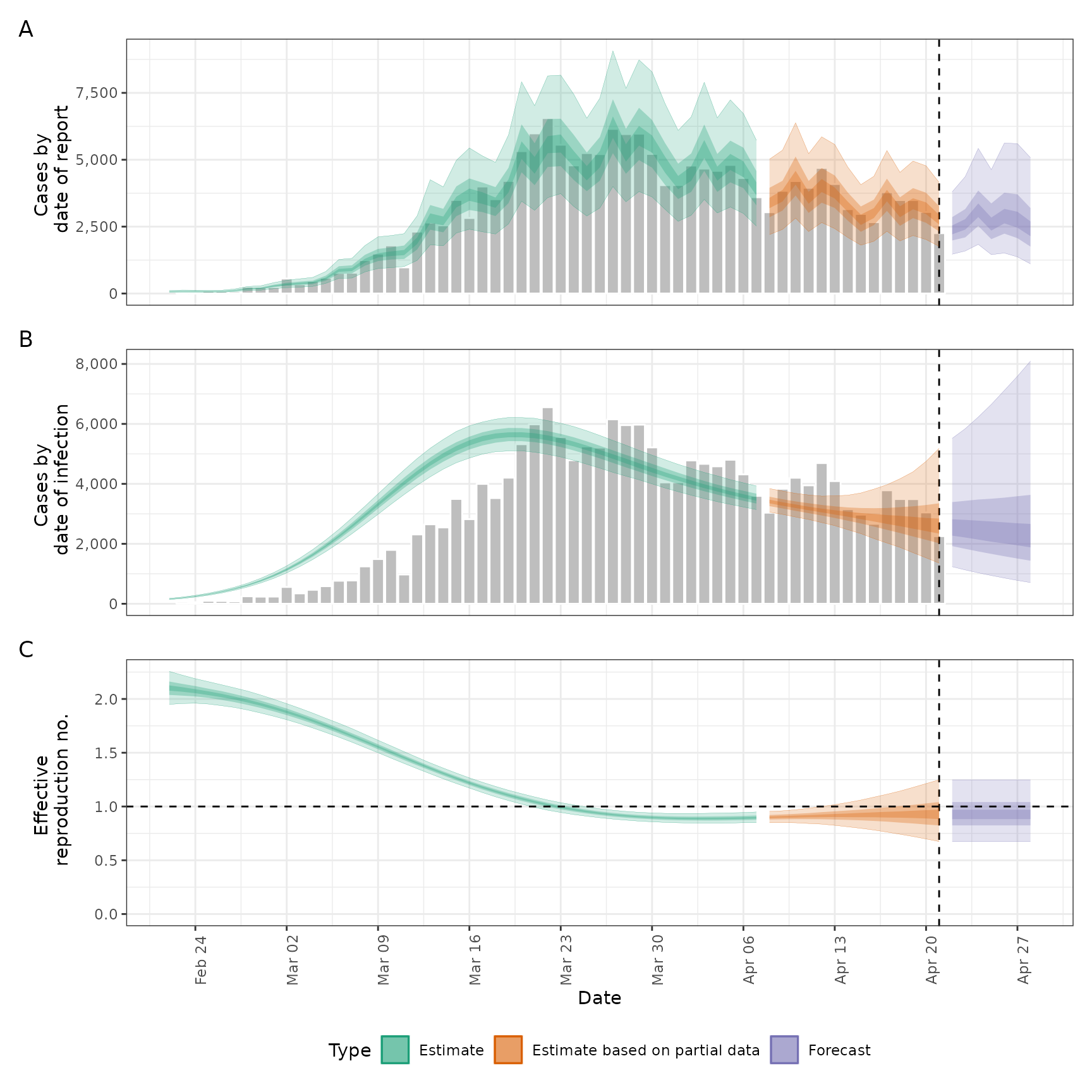# using back calculation (combined here with under reporting)
# this model is in the order of 10 ~ 100 faster than the gaussian process
# method
# it is likely robust for retrospective Rt but less reliable for real time
# estimates
# the width of the prior window controls the reliance on observed data and
# can be optionally switched off using backcalc_opts(prior = "none"),
# see ?backcalc_opts for other options
backcalc <- estimate_infections(reported_cases,
generation_time = generation_time_opts(generation_time),
delays = delay_opts(incubation_period + reporting_delay),
rt = NULL, backcalc = backcalc_opts(),
obs = obs_opts(scale = list(mean = 0.4, sd = 0.05)),
horizon = 0
)
plot(backcalc)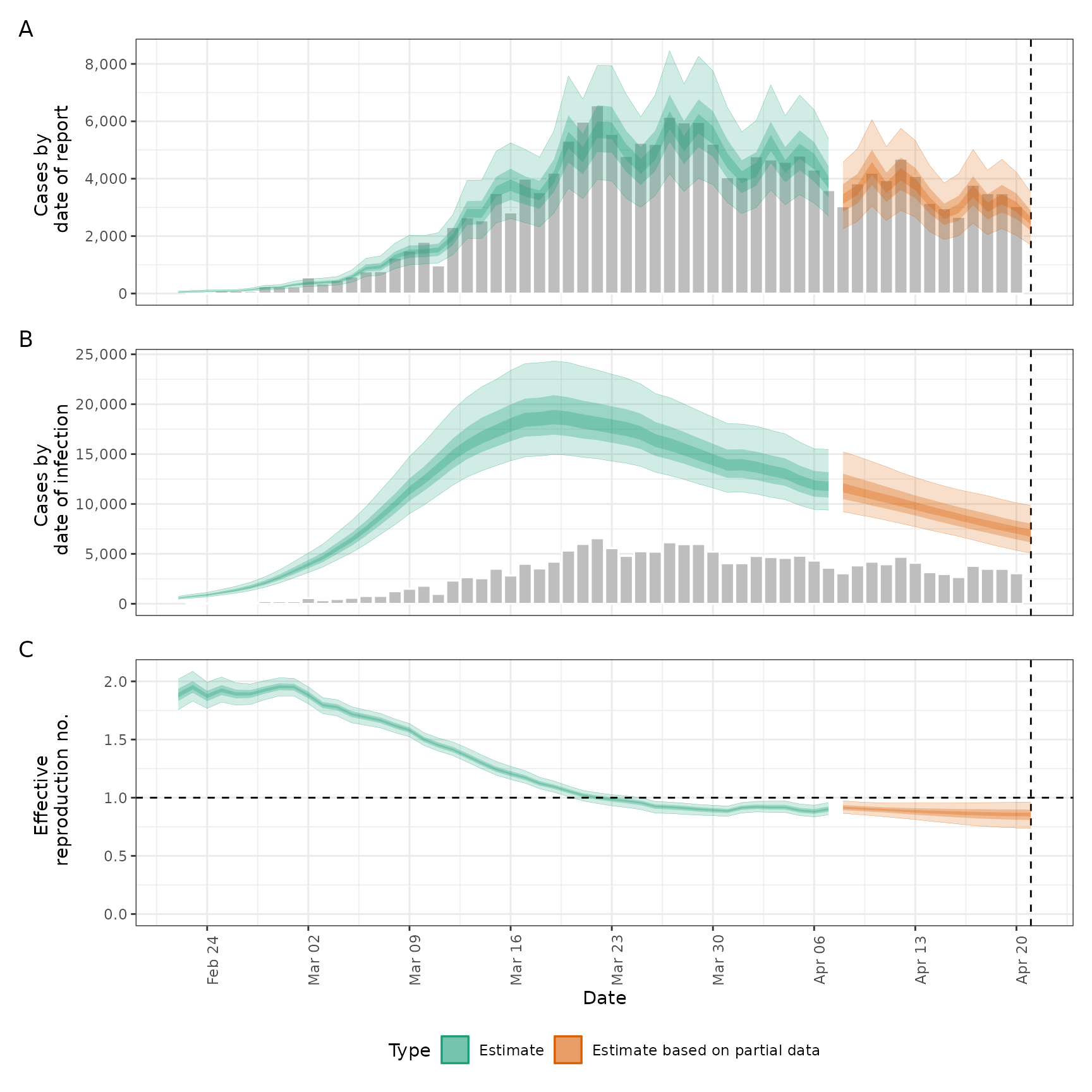# Rt projected into the future using the Gaussian process
project_rt <- estimate_infections(reported_cases,
generation_time = generation_time_opts(generation_time),
delays = delay_opts(incubation_period + reporting_delay),
rt = rt_opts(
prior = list(mean = 2, sd = 0.1),
future = "project"
)
)
#> Warning: There were 2 divergent transitions after warmup. See
#> https://mc-stan.org/misc/warnings.html#divergent-transitions-after-warmup
#> to find out why this is a problem and how to eliminate them.
#> Warning: Examine the pairs() plot to diagnose sampling problems
plot(project_rt)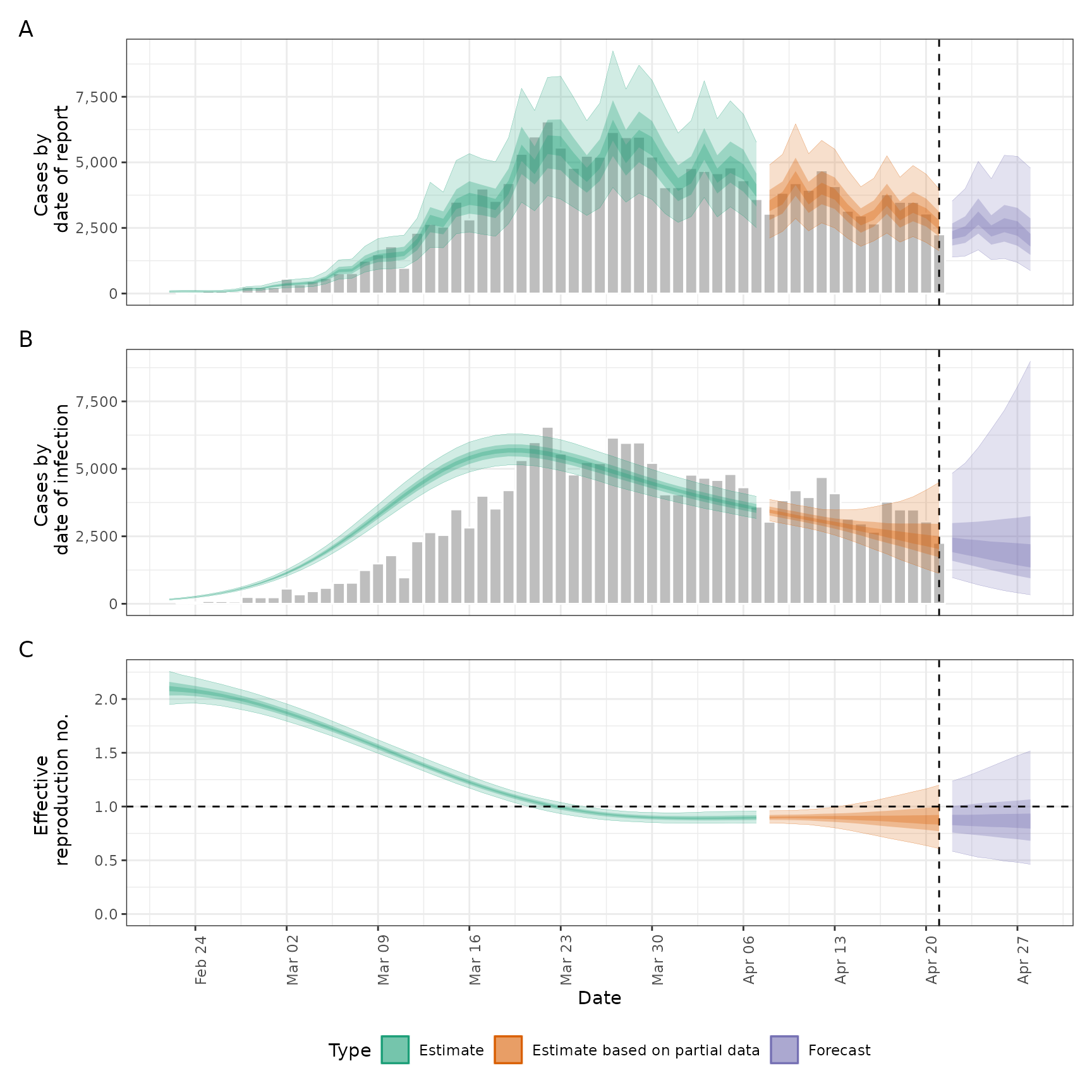# default settings on a later snapshot of data
snapshot_cases <- example_confirmed[80:130]
snapshot <- estimate_infections(snapshot_cases,
generation_time = generation_time_opts(generation_time),
delays = delay_opts(incubation_period + reporting_delay),
rt = rt_opts(prior = list(mean = 1, sd = 0.1))
)
#> Warning: There were 9 divergent transitions after warmup. See
#> https://mc-stan.org/misc/warnings.html#divergent-transitions-after-warmup
#> to find out why this is a problem and how to eliminate them.
#> Warning: Examine the pairs() plot to diagnose sampling problems
plot(snapshot)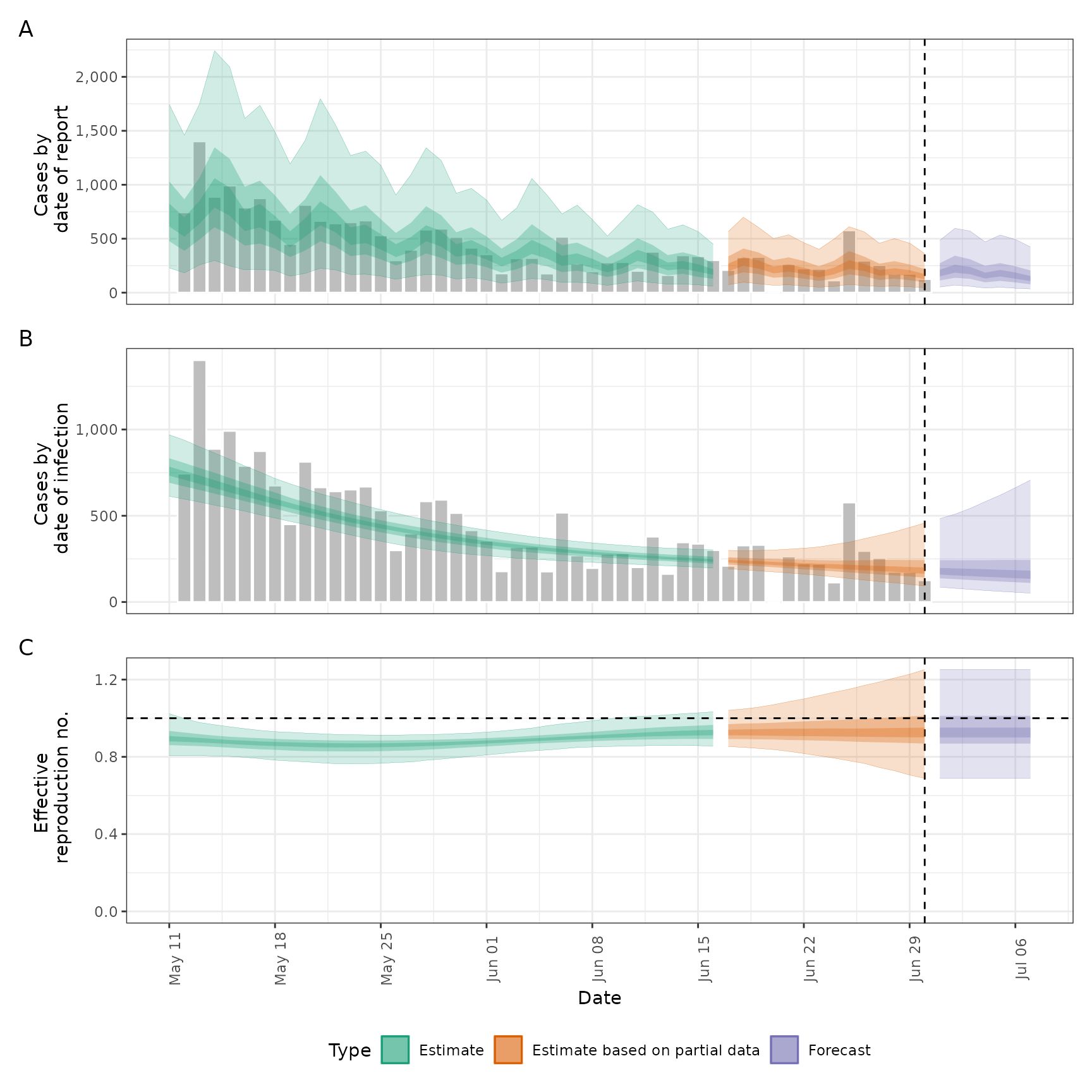# stationary Rt assumption (likely to provide biased real-time estimates)
# with uncertain reporting delays
stat <- estimate_infections(reported_cases,
generation_time = generation_time_opts(generation_time),
delays = delay_opts(incubation_period_uncertain + reporting_delay),
rt = rt_opts(prior = list(mean = 2, sd = 0.1), gp_on = "R0")
)
plot(stat)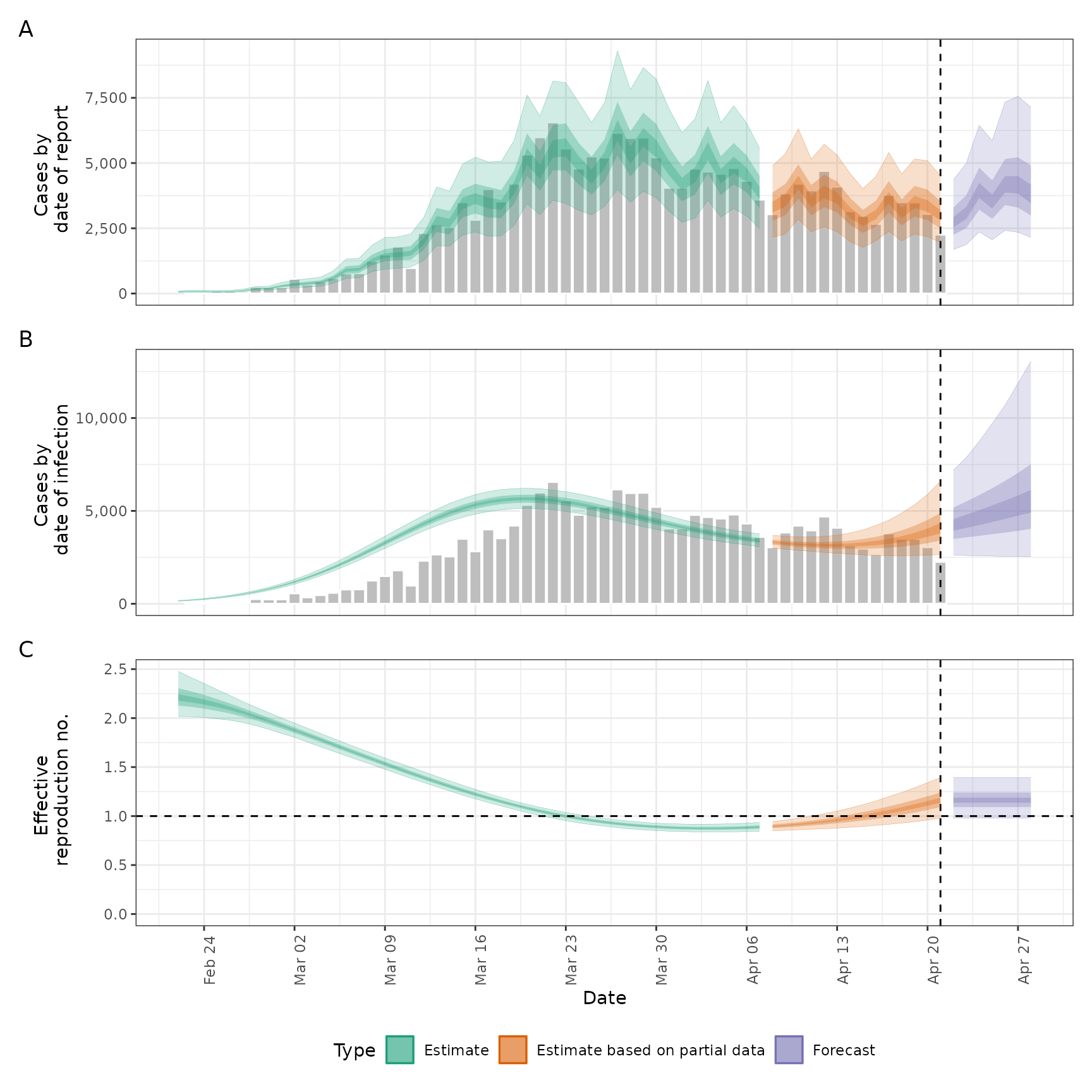# no gaussian process (i.e fixed Rt assuming no breakpoints)
# with uncertain reporting delays
fixed <- estimate_infections(reported_cases,
generation_time = generation_time_opts(generation_time),
delays = delay_opts(incubation_period_uncertain + reporting_delay),
gp = NULL
)
plot(fixed)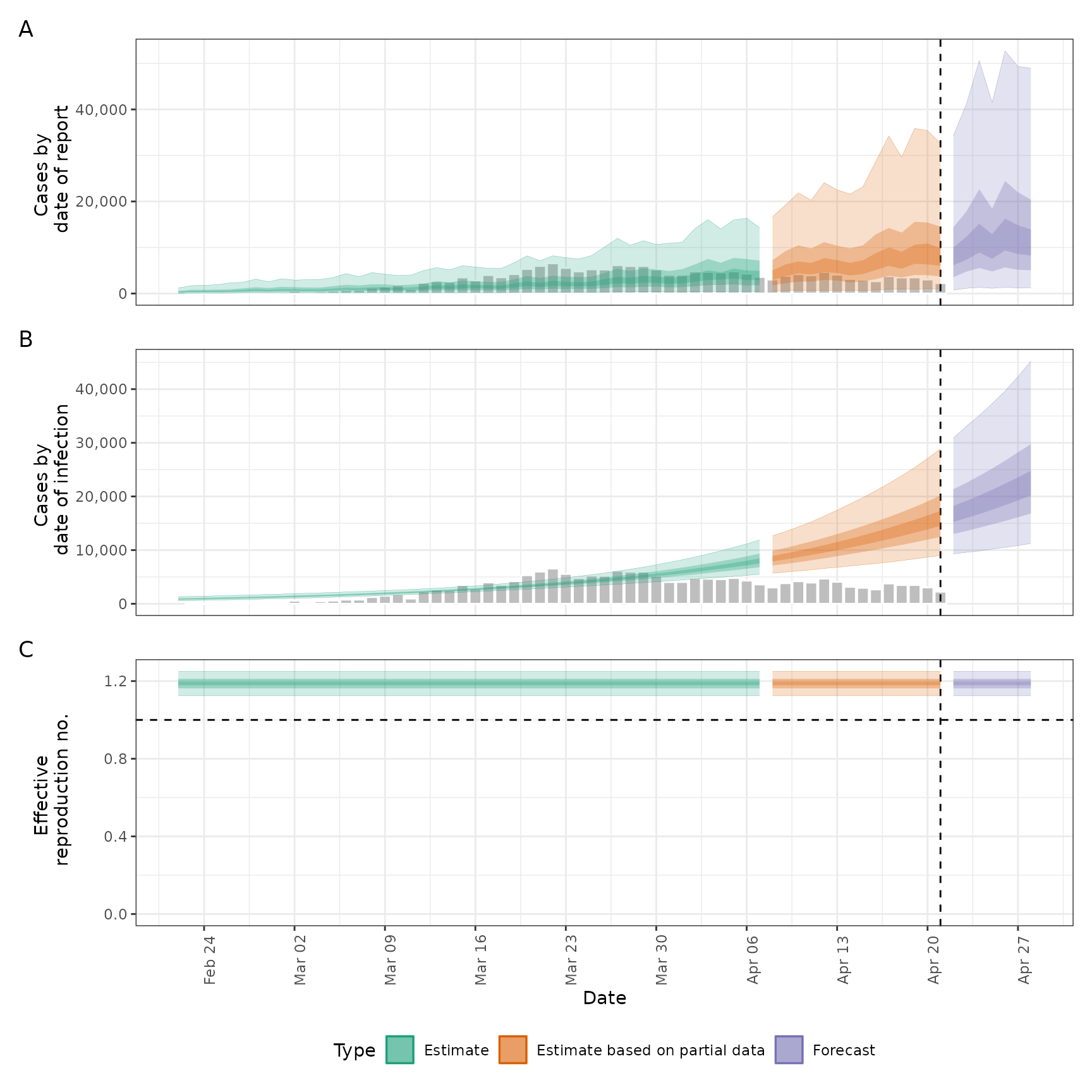# no delays
no_delay <- estimate_infections(
reported_cases,
generation_time = generation_time_opts(generation_time)
)
#> Warning: There were 6 divergent transitions after warmup. See
#> https://mc-stan.org/misc/warnings.html#divergent-transitions-after-warmup
#> to find out why this is a problem and how to eliminate them.
#> Warning: Examine the pairs() plot to diagnose sampling problems
plot(no_delay)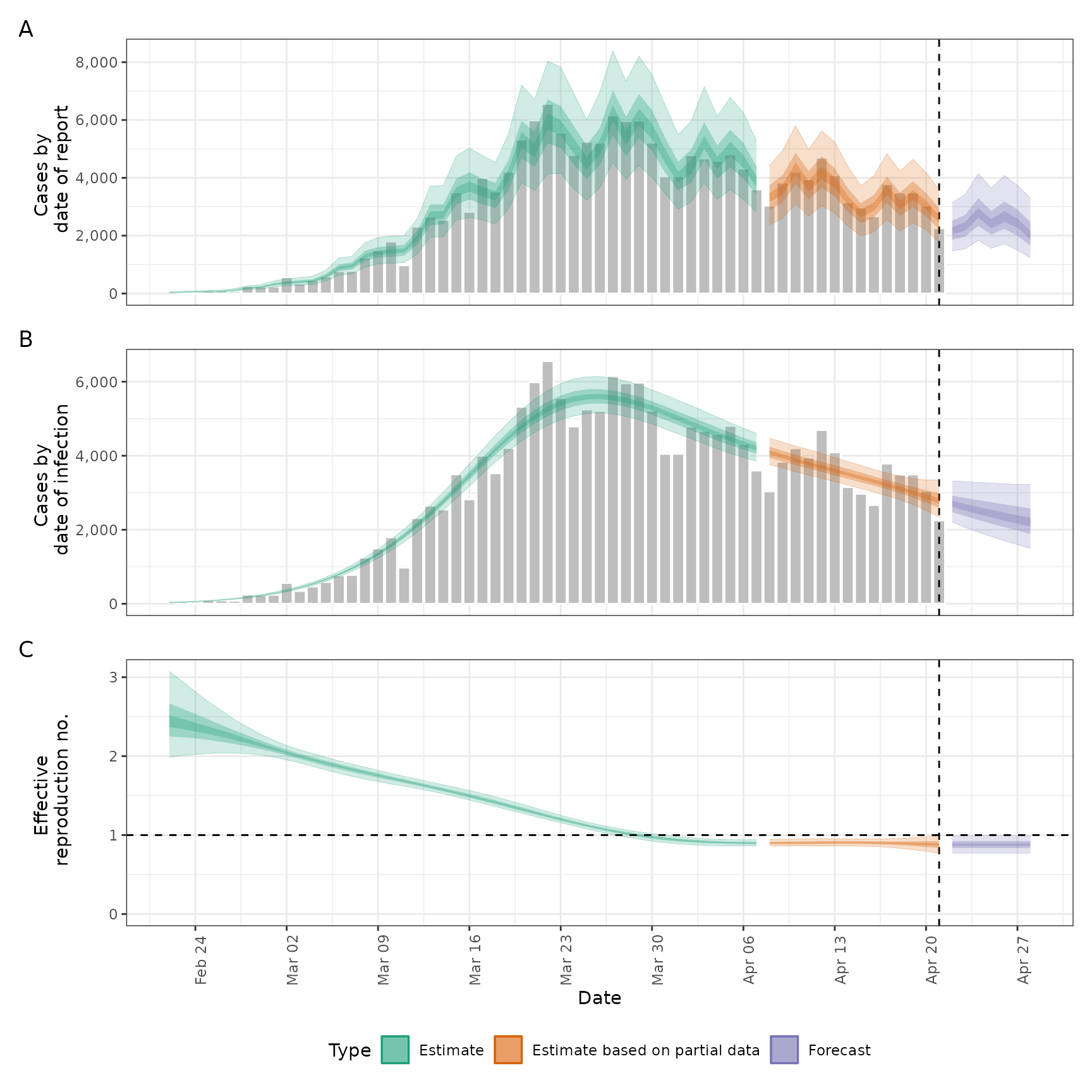# break point but otherwise static Rt
# with uncertain reporting delays
bp_cases <- data.table::copy(reported_cases)
bp_cases <- bp_cases[,
breakpoint := ifelse(date == as.Date("2020-03-16"), 1, 0)
]
bkp <- estimate_infections(bp_cases,
generation_time = generation_time_opts(generation_time),
delays = delay_opts(incubation_period_uncertain + reporting_delay),
rt = rt_opts(prior = list(mean = 2, sd = 0.1)),
gp = NULL
)
# break point effect
summary(bkp, type = "parameters", params = "breakpoints")
#>    date    variable strat type     median       mean         sd   lower_90
#> 1: <NA> breakpoints     1 <NA> -0.6706079 -0.6707402 0.03079727 -0.7205391
#>      lower_50  lower_20  upper_20   upper_50   upper_90
#> 1: -0.6920782 -0.677934 -0.663612 -0.6496607 -0.6199435
plot(bkp)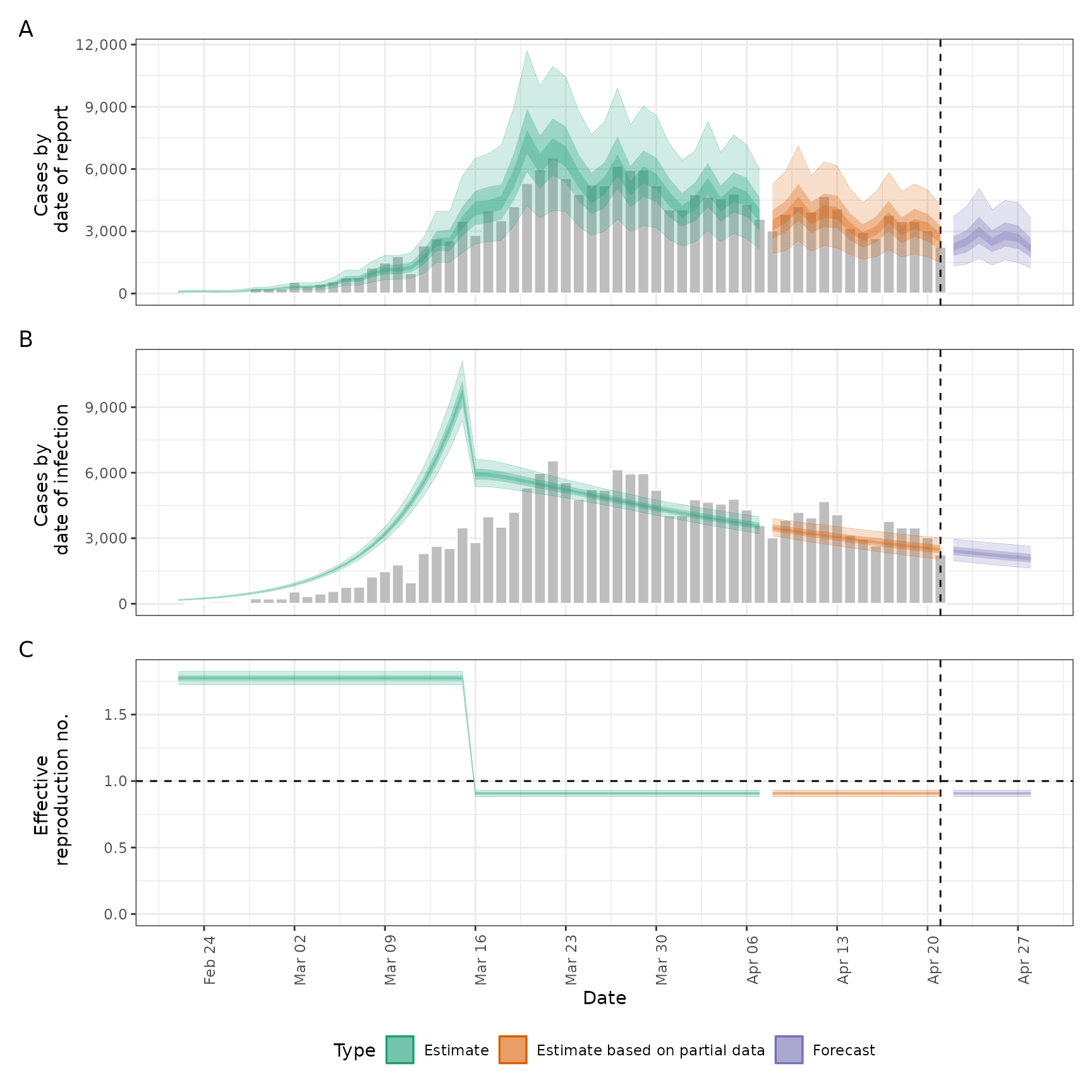# weekly random walk
# with uncertain reporting delays
rw <- estimate_infections(reported_cases,
generation_time = generation_time_opts(generation_time),
delays = delay_opts(incubation_period_uncertain + reporting_delay),
rt = rt_opts(prior = list(mean = 2, sd = 0.1), rw = 7),
gp = NULL
)

# random walk effects
summary(rw, type = "parameters", params = "breakpoints")
#>    date    variable strat type      median        mean         sd   lower_90
#> 1: <NA> breakpoints     1 <NA> -0.13147171 -0.13165406 0.06754508 -0.2436721
#> 2: <NA> breakpoints     2 <NA> -0.16519498 -0.16579733 0.08090044 -0.2985345
#> 3: <NA> breakpoints     3 <NA> -0.22426607 -0.22009731 0.09409975 -0.3700840
#> 4: <NA> breakpoints     4 <NA> -0.27288529 -0.27893416 0.10131415 -0.4519362
#> 5: <NA> breakpoints     5 <NA> -0.06125400 -0.06214609 0.10181322 -0.2285185
#> 6: <NA> breakpoints     6 <NA>  0.03729778  0.03622026 0.10364271 -0.1322581
#> 7: <NA> breakpoints     7 <NA> -0.05214400 -0.05366692 0.11556742 -0.2430230
#> 8: <NA> breakpoints     8 <NA> -0.01826475 -0.02225903 0.17383430 -0.3114837
#>       lower_50    lower_20    upper_20     upper_50    upper_90
#> 1: -0.17719182 -0.14870718 -0.11245775 -0.084840789 -0.02463561
#> 2: -0.21731991 -0.18472591 -0.14526880 -0.112817291 -0.03270595
#> 3: -0.28347439 -0.24447000 -0.19886899 -0.156702712 -0.06781363
#> 4: -0.34821974 -0.30158352 -0.24941575 -0.205805213 -0.11860007
#> 5: -0.13155188 -0.08593423 -0.03672112  0.003281974  0.10832854
#> 6: -0.03459092  0.01008262  0.06048472  0.102449845  0.20412552
#> 7: -0.12453964 -0.07880822 -0.02389124  0.024575211  0.13557993
#> 8: -0.13592836 -0.06196672  0.02553465  0.091020111  0.25225923
plot(rw)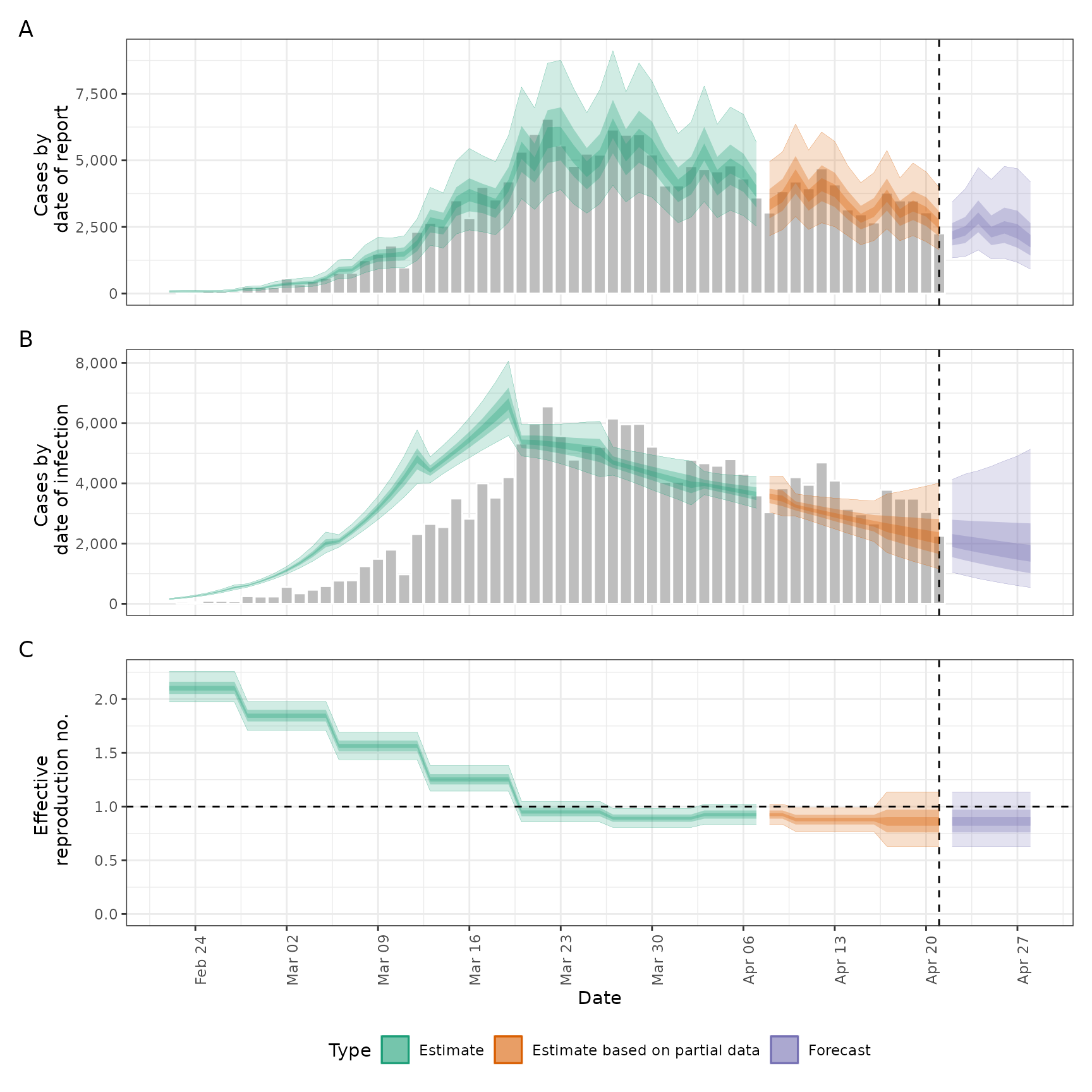options(old_opts)
# }